Partial Residuals and the termplot function

Earlier this year I taught an Intro to R Graphics workshop. While preparing for it I came across the termplot function. I had no idea it existed. Of course now that I know about it, I come across it fairly regularly. (That's the Baader-Meinhof Phenomenon for you.)

?termplot will tell you what it does: “Plots regression terms against their predictors, optionally with standard errors and partial residuals added.” If you read on, you'll see “Nothing sensible happens for interaction terms, and they may cause errors.” Duly noted.

Let's try this out and see what it does.

First we'll fit a model using the mtcars dataset that comes with R. Below mpg = Miles/(US) gallon, drat = Rear axle ratio, qsec = ¼ mile time, disp = Displacement (cu.in.), and wt = Weight (lb/1000).

lm.cars <- lm(mpg ~ wt + disp + drat + qsec, data=mtcars)
summary(lm.cars)
##
## Call:
## lm(formula = mpg ~ wt + disp + drat + qsec, data = mtcars)
##
## Residuals:
##    Min     1Q Median     3Q    Max
## -4.143 -1.933 -0.149  0.919  5.541
##
## Coefficients:
##             Estimate Std. Error t value Pr(>|t|)
## (Intercept)  9.03223    9.58829    0.94  0.35454
## wt          -4.86768    1.20872   -4.03  0.00041 ***
## disp         0.00522    0.01105    0.47  0.64021
## drat         1.87086    1.32462    1.41  0.16926
## qsec         1.05248    0.34778    3.03  0.00539 **
## ---
## Signif. codes:  0 '***' 0.001 '**' 0.01 '*' 0.05 '.' 0.1 ' ' 1
##
## Residual standard error: 2.6 on 27 degrees of freedom
## Multiple R-squared:  0.838,  Adjusted R-squared:  0.814
## F-statistic:   35 on 4 and 27 DF,  p-value: 2.55e-10
par(mfrow=c(2,2))
termplot(lm.cars)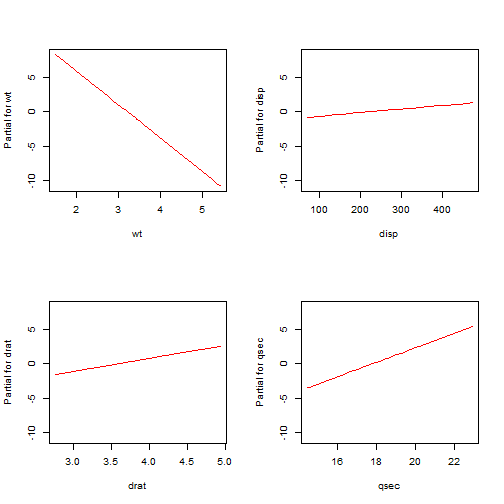Compare the coefficients in the summary output to the slopes of the lines in the graphs. See how the coefficients match the slopes of the lines? That's because they are the slopes of the lines. For example, wt has a coefficient of about -5. Likewise the termplot for that coefficient has a negative slope that appears to be about -5. Using these plots we can see at a glance the respective contributions of the predictors. wt and qsec seem to contribute to the model due to the steepness of their slopes, but disp and drat not so much. This matches the summary output that shows wt and qsec as being significant.

Now termplot also has an argument called partial.resid that will add partial residuals if set to TRUE. Let's try that out, with color set to “purple” since the default gray can be hard to see (at least to my eyes):

par(mfrow=c(2,2))
termplot(lm.cars, partial.resid=TRUE, col.res = "purple")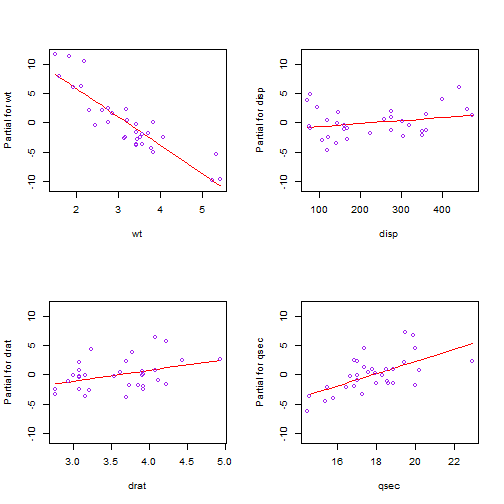How are these points generated? The x-axis is clear enough. That's just the predictor values. But the y-axis says “Partial for [variable name]”, i.e. partial residuals. What does that mean? Put as simply as possible, partial residuals are the sum of the residuals and predictor terms. Hopefully you know what residuals are. Those are just the difference between the observed and fitted response values. “Predictor terms” is a little trickier.

To understand them, it helps to know that

$y = \bar{y} + b_{1}(x_{1} – \bar{x}_{1}) + b_{2}(x_{2} – \bar{x}_{2}) + b_{3}(x_{3} – \bar{x}_{3}) + b_{4}(x_{4} – \bar{x}_{4}) + e$

is another way of writing

$y = b_{0} + b_{1}x_{1} + b_{2}x_{2}+ b_{3}x_{3}+ b_{4}x_{4} + e$

So the first equation above has $$\bar{y}$$ as the intercept and centered predictors. If we center the predictors and fit a model, we'll see that's precisely what we get:

# center the variables
mtcars <- transform(mtcars, wtC = wt-mean(wt), dispC = disp-mean(disp),
dratC=drat-mean(drat), qsecC=qsec-mean(qsec))
# now fit model using centered predictors
lm.terms.cars <- lm(mpg ~ wtC + dispC + dratC + qsecC, data=mtcars)
coef(lm.terms.cars)
## (Intercept)
##       20.09
mean(mtcars$mpg) ##  20.09 Now if we take the centered predictor values and multiply them by their respective coefficients, we get what are referred to in R as terms. For example, the terms for the first record in the data set are as follows: # take all coefficients but the intercept... coef(lm.terms.cars)[-1] ## wtC dispC dratC qsecC ## -4.867682 0.005222 1.870855 1.052478 # ...and first record of data... lm.terms.cars$model[1,-1]
##               wtC  dispC  dratC  qsecC
## Mazda RX4 -0.5972 -70.72 0.3034 -1.389
# ...and multiply:
coef(lm.terms.cars)[-1]*t(lm.terms.cars$model[1,-1]) ## Mazda RX4 ## wtC 2.9072 ## dispC -0.3693 ## dratC 0.5677 ## qsecC -1.4616 So for the first record, the term due to wt is 2.91, the term due to disp is -0.37, and so on. These are the predictor terms I mentioned earlier. These are what we add to the residuals to make partial residuals. You'll be happy to know we don't have to center predictors and fit a new model to extract terms. R can do that for us on the original model object when you specify type="terms" with the predict function, like so: # notice we're calling this on the original model object, lm.cars pterms <- predict(lm.cars, type="terms") pterms[1,] # compare to above; the same ## wt disp drat qsec ## 2.9072 -0.3693 0.5677 -1.4616 To calculate the partial residuals ourselves and make our own partial residual plots, we can do this: # add residuals to each column of pterms (i.e., the terms for each predictor) partial.residuals <- apply(pterms,2,function(x)x+resid(lm.cars)) # create our own termplot of partial residuals par(mfrow=c(2,2)) # the model in lm.cars includes the response in first column, so we index with i + 1 for(i in 1:4){ plot(x=lm.cars$model[,(i+1)],y=partial.residuals[,i], col="purple", ylim=c(-10,10))
abline(lm(partial.residuals[,i] ~ lm.cars$model[,(i+1)]), col="red") }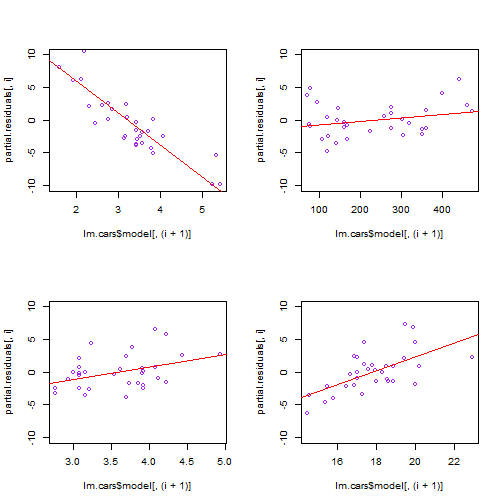So that's what termplot does for us: it takes the terms for each predictor, adds the residuals to the terms to create partial residuals, and then plots partial residuals versus their respective predictor, (if you specify partial.resid=TRUE). And of course it plots a fitted line, the result of regressing the predictor's partial residuals on itself. Recall ealier I mentioned the slope of the line in the termplot graphs is the coefficient in the summary output. We can indeed verify that: # coefficient for wt coef(lm.cars) ## wt ## -4.868 coef(lm(partial.residuals[,1] ~ mtcars$wt))
## mtcars$wt ## -4.868 Ultimately what this whole partial residual/termplot thing is doing is splitting the response value into different parts: an overall mean, a term that is due to wt, a term that is due to disp, a term that is due to drat, and a term that is due to qsec. And you have the residual. So when we create the partial residual for wt, what we're doing is adding the wt term and the residual. This sum accounts for the part of the response not explained by the other terms. Again let's look at the overall mean, the terms for the first observation and its residual: # overall mean of response mean(mtcars$mpg)
##  20.09
# terms of first observation
pterms[1,]
##      wt    disp    drat    qsec
##  2.9072 -0.3693  0.5677 -1.4616
# the residual of the first observation
resid(lm.cars)
## Mazda RX4
##   -0.7346

If we add those up, we get the observed value

# add overall mean, terms and residual for first observation
mean(mtcars$mpg) + sum(pterms[1,]) + resid(lm.cars) ## Mazda RX4 ## 21 # same as observed in data mtcars$mpg
##  21

So the wt term value of 2.9072 represents the part of the response that is not explained by the other terms.

Finally, we can add another argument to termplot, smooth=panel.smooth that will draw a smooth lowess line through the points. This can help us assess departures from linearity.

par(mfrow=c(2,2))
termplot(lm.cars, partial.resid=TRUE, col.res = "purple", smooth=panel.smooth)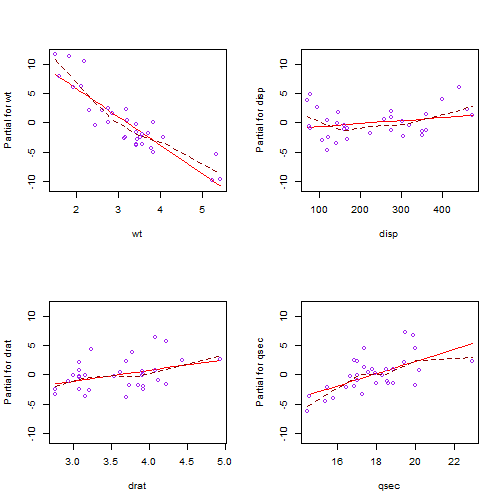Notice how the dashed line bends around the straight line for wt. Perhaps wt requires a quadratic term? Let's try that using the poly function, which creates orthogonal polynomials.

lm.cars2 <- lm(mpg ~ poly(wt,2) + disp + drat + qsec, data=mtcars)
par(mfrow=c(2,2))
termplot(lm.cars2, partial.resid=TRUE, col.res = "purple", smooth=panel.smooth)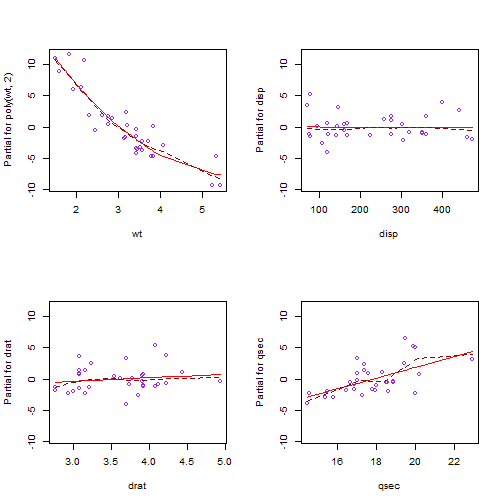We now see a better fit with the regressed line matching closely to the smooth line.